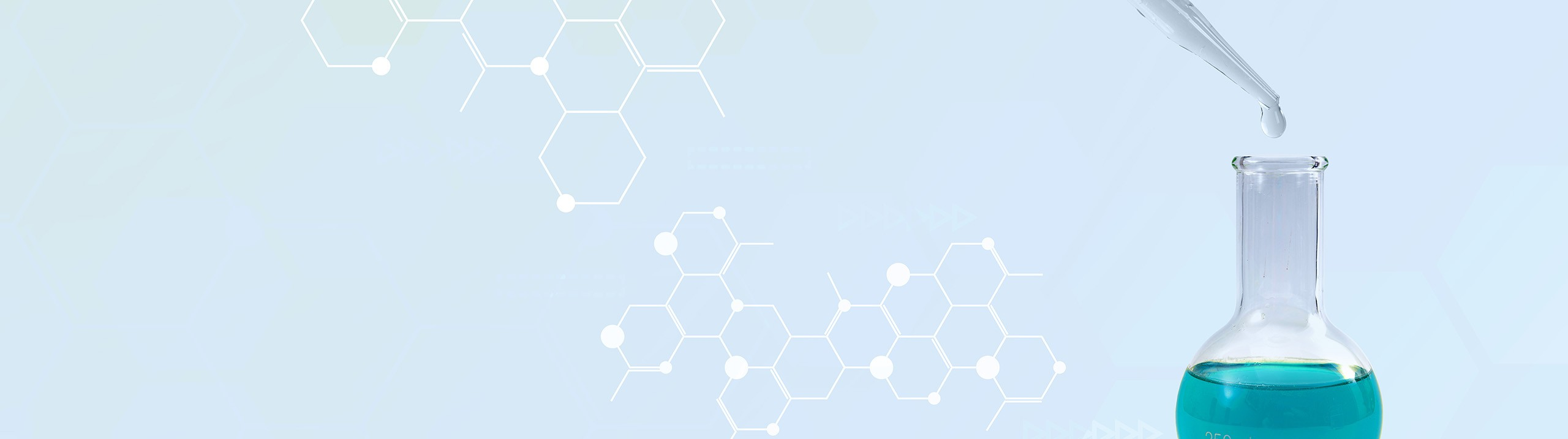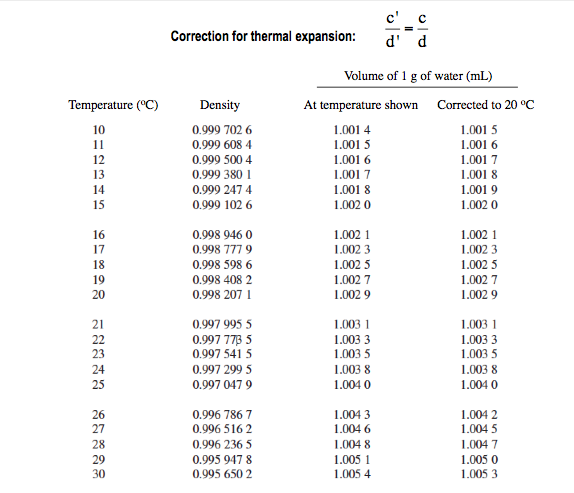Start typing, then use the up and down arrows to select an option from the list.# Analytical Chemistry

Learn the toughest concepts covered in your Analytical Chemistry class with step-by-step video tutorials and practice problems.

# Thermal Dependency

## Thermal Expansion

1

#### concept

Thermal Expansion

The video is coming soon
with every calculation and measurement, we have to be sure to minimize all outside factors so that our data isn't corrupted. Now one of the outside factors we have to be in mind of is thermal dependency. Now we're gonna say the process of calibration is the process of measuring the actual quantity of mass, volume or other type of of chemical measurements that relate to what we observe on an analytical scale. So again we're trying to be as accurate and precise as possible with all our calculations so we have to make sure that we eliminate or at least try to minimize as much as possible any outside factors. Now, the idea of temperature comes into play here, we're gonna say in general when taking the measurements of a solution, we have to take into account any type of thermal expansion that could somehow influence either our solution or our instrumentation. So the temperature of the room, the temperature of the instruments themselves can have an effect on the volume that's being recorded with every type of um lab process that we're undergoing. Now we're gonna say here we can correct for this thermal expansion by using our formula. So here we're gonna say that C. Prime represents the concentration of our solution divided by D. Prime which is the density of our solution. It equals the new concentration divided by the new density of our solution. Now if we take a look here We look at this graph in this graph, we have the temperature of our solution or temperature of water at different numbers all the way from 10°C to 30°C. Now, if you take a look as we're going from 10-30, we can see that our temperature is clearly increasing. Now look at the density of water as the temperature changes as it increases, what's happening to our density while we're starting up here with 0.99970 to six. And as you go down, you should notice that the density is decreasing as our temperature is increasing later on, we'll talk about why this is so, but just remember for right now that the trend is, as our temperature is increasing, our density will decrease The next two columns. So here, what we have is at temperature shown. So we're recording the volume of a container or recording the volume from some type of instrument at a given temperature. This would be the volume of the water for every one g. So let's say that we're taking uh volumetric analysis of water delivered at 10°C, we'd say at 10°C, we would basically deliver 1.14 mls of water for every one g of water. But here's the thing, when it comes to our instruments, all the glassware is usually calibrated to around 20 degrees Celsius. So here we have corrected the volume delivered to 20 degrees Celsius because that's the temperature in which we calibrate our glassware. So in actuality it's really delivering 1.15 mls for every one g of water. So remember the column on the left? This column here is a temperature um the volume record when we know a specific temperature. And this is us correcting for it based on the calibration temperature of most glass wares within your analytical lab. Now keep in mind this chart as we go and venture off into thermal expansion. No, you don't have to memorize all the numbers. Just remember the general trends that are associated with it. If you have questions dealing with this, you would be given values in some way or another. Either through the question itself or given this chart on a separate sheet of paper. So guys, now that we've covered this, move on to the example that's given on the bottom, see if you can tackle that question which relates polarity with parts Pavilion and also talks about this idea of thermal expansion. What effect does it have on the polarity Once you've done that, come back and see how I approach the same exact question2

#### example

Thermal Expansion

The video is coming soon

## Thermal Expansion Calculations

3

#### example

Thermal Dependency Calculations3m
Play a video: